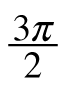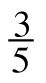Home > CCA2 > Chapter 12 > Lesson 12.2.1 > Problem12-100

12-100.

If πθand sin θ = −, find cos θ. Use what you know about the Pythagorean Identity and the unit circle to get started. Homework Help ✎Remember that the Pythagorean Identity is sin²(θ) + cos²(θ) = 1.
Try substituting the given value of sin(θ) into the equation, then solve for cos(θ).

(−3/5)2 + cos2(θ) = 1

cos2(θ) = 16/25

Take the square root of both sides to solve for cos (θ).
Now use the unit circle to decide what the correct sign of the answer is.